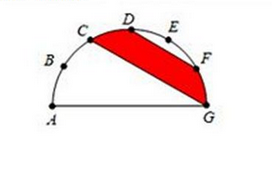# It may look complicated, but it's not!

Geometry Level 2The diagram above shows a semicircle with $A,B,C,D,E,F$ and $G$ are on its circumference with $\widehat{AB}=\widehat{BC}=\widehat{CD}=\widehat{DE}=\widehat{EF}=\widehat{FG}$. Find the area of the red filled area, given that the area of the semicircle is $60\text{ cm}^2$.

×